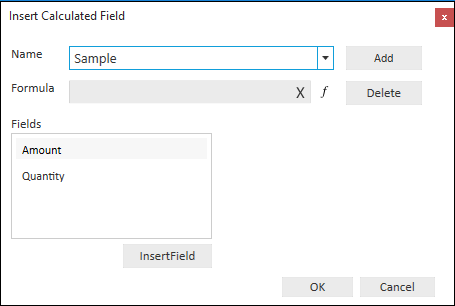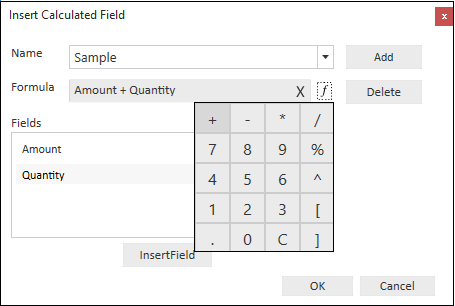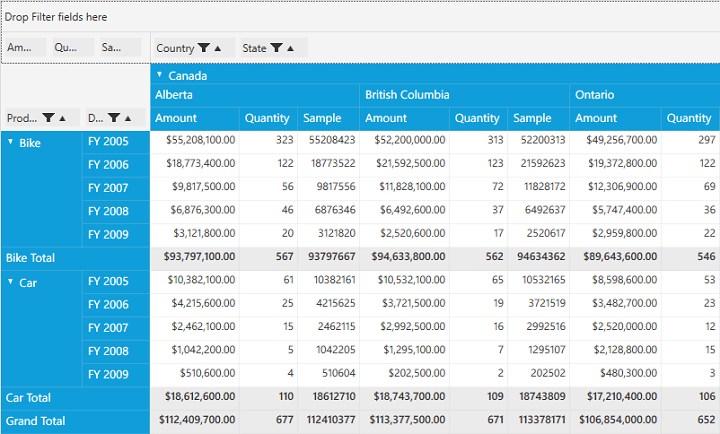# Calculated Field in WPF Pivot Grid

The pivot grid inserts a new calculated field based on the existing calculated items using the calculated field window. This option is available as a menu item in the grouping bar context menu.

Inserting a new calculated field in pivot grid

To insert a new calculated field, open the calculated field window using the grouping bar context menu. Then, define a Name for the new calculated field.Defining Name for the Calculated Field

Note that, the Formula can be entered by inserting calculation fields through the Fields section. For inserting numerical operator, you can use the formula pop-up as shown in the following screenshot.Entering Formula for the Calculated Field

Click Add to add the calculated field and OK to populate the pivot grid control.PivotGrid with newly added Calculated Field